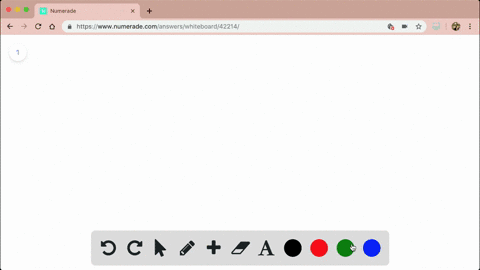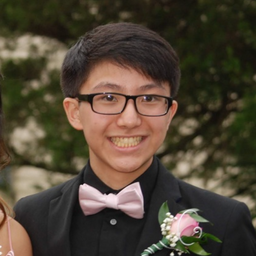🎉 The Study-to-Win Winning Ticket number has been announced! Go to your Tickets dashboard to see if you won! 🎉View Winning Ticket### Evaluate each double integral. If the function se…

03:27Rutgers, The State University of New Jersey
Problem 57

# Evaluate each double integral. If the function seems too difficult to integrate, try interchanging the limits of integration, as in Exercises 37 and \$38 .$$\int_{0}^{\ln 2} \int_{e^{x}}^{2} \frac{1}{\ln x} d x d y$$

## Discussion

You must be signed in to discuss.

## Video Transcript

All right. So we're giving a double. Integral in this form, integral from Syria to Elena, too, of the interval from Eades eats the power of wine to two of the function. One over Ellen of X dxc. Why? And we're told to evaluate this double inter goal into an exploits. Explicit solution. Um, and the weird thing here is what you're placing to yourself is, um what the heck, I even know how toe integrate Ellen of X. And now it's in the denominator, So I totally don't know how. Toe, um, integrate this and get explosive solution on. Um, I'm here to tell you that you're right. I I don't know either. Um, but there is a worker way around this, and it just involves switching the on order of integration. So instead of doing a dxc y integral, we're actually going to use a d y d x integral. Um, but we can't just willy nilly just switch the limits like Syria to to oser, to Ellen and to, um, and use the y two. We can't do that because then this kind of changes up what our region looks like, Um, that we're integrating over. So We need to be a little bit careful and not hesitantly or naively do that. So let's take it from a more, um, a smarter approach, the correct way. So, um, I guess our first question is, what what are these Limb is actually saying Tow us. Um, the first thing I say to myself is, Okay, we have this inner integral, and it's saying that since we're integrating spent two x x ranges from this function to this number. So what we're saying is X ranges from X equals each of the why two X equals two. Okay. And then why ranges from why equals zero to body equals Eleanor too. Okay. And let's kind of just grab this out to see what it looks like. Um, we have y equals zero, obviously, right here, um, X equals two right here. And then let's deal with our other odd numbers. So x e to the y may look a little weird to graph, but let's just, uh, kind of just rearrange what it looks like. I'm just gonna ply and Ellen to both sides, and this should just result in why equals Ellen affects, and we kind of know what Ellen of X looks like. If you remember, it kind of looks like this. This is why equals Allen of X. Okay. And it passes through Ah, 10 right here. Because if you plug in one for X, basically, you're asking yourself what exponents gives you, uh, raised to what power gives you one, and it should just be zero. So basically ah, you have your coordinate right here. 10 and then you have basically after you draw that out. Um oh, we have also this founding thing, but actually intersex right here y equals Ellen of to. And that's because they're basically they all intersect right here. Because if you plug in to for Ln you get Elena to. So basically, this corner right here is to Ellen to so actually works out perfectly perfectly. Just, um, creates this little, uh, round triangle thing. But now we can have a sense of what our region are looks like and we kind of we can now rewrite our double integral to be a d y the X integral in the correct way. So let's think about first how? Why ranges, Um and what I like to do in my head. This is what I do usually in my head, Um, kind of think like I draw like a vertical line in my head, and then I draw dot line and then see it pass out. So basically, um, I say it enters that y equals zero are basically is bounded by white will serve as its lowered around. So the lower bound is Europe, and then it leaves this region at y equals L Innovex. So Elena vexes our upper bound. So why basically ranges from zero to l Innovex and then we want to say, how how long does this occur? Or basically, for what I sell use is, um, our bounds y equals zero and Ellen Vex, and that should give us the X limits. And as you can see, uh uh, X equals 12 X equals two are the limits that why ranges in this manner. So we have these limits, okay? And now we can take a better approach at solving this political. And let's just do solve the inner integral first. So we have this, um, so we can, since there's no wise in here. Basically, this is a whole just entire constant. So we can just, um, take it out of the integral like so we have just one d y. And I'm not sure if you guys know yet that, um basically the inter goal of the function one, the single integral of the function One is basically just saying What's the length of our interval? So basically, you just be l a next minus zero and that's just Ellen X. So this whole integral just evaluates to uh l Innovex. And you could just verify that if you want to try integrating your way, you would just have this being why, from zero to Alan Vex and you would see it just Ellen vax minus zero. And it just basically, it's always gonna be the length of the interval because the single interval of one just tells you the length of the interval. Dyker integrating over and that does journal eyes to hire number of inter girls. But you'll probably learn that a little bit later are actually pretty soon anyways, so this is the value. So this should just evaluate toe one, which is perfect, because then we can apply it to the other and will want to of won t x And we just learned that the single integral of the function one is basically just the length of the interval and our length of the length of this interval is just one. So that is our final answer.

## Recommended Questions

#### You're viewing a similar answer. To request the exact answer, fill out the form below:

Our educator team will work on creating an answer for you in the next 6 hours.